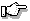# fcvt(), _fcvt()

convert a floating-point number into a string

## Synopsis:

```#include <stdlib.h>
char *fcvt( double value,
int ndigits,
int *dec,
int *sign );
char *_fcvt( double value,
int ndigits,
int *dec,
int *sign );```

## Description:

The fcvt() function converts the floating-point number value into a character string. The parameter ndigits specifies the number of digits desired after the decimal point. The converted number will be rounded to this position.

The character string will contain only digits, and is terminated by a NULL character. The integer pointed to by dec will be filled in with a value indicating the position of the decimal point, relative to the start of the string of digits. A zero or negative value indicates that the decimal point lies to the left of the first digit. The integer pointed to by sign will contain 0 if the number is positive, and non-zero if the number is negative.

The _fcvt() function is identical to fcvt(). Use _fcvt() for ANSI/ISO naming conventions.

## Returns:

A pointer to a static buffer containing the converted string of digits.ecvt() and fcvt() both use the same static buffer.

## Examples:

```#include <stdio.h>
#include <stdlib.h>

void main()
{
char *str;
int  dec, sign;

str = fcvt( -123.456789, 5, &dec, &sign );
printf( "str=%s, dec=%d, sign=%d\n", str, dec,
sign );
}```

produces the output:

`str=12345679, dec=3, sign=-1`

## Classification:

WATCOM

_fcvt() conforms to ANSI/ISO naming conventions.

Safety:
Interrupt handler No
Signal handler No